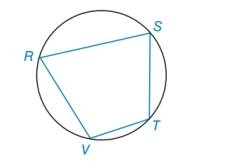Chapter 6.2, Problem 19EElementary Geometry For College St...

7th Edition
Alexander + 2 others
ISBN: 9781337614085

Solutions

Chapter
SectionElementary Geometry For College St...

7th Edition
Alexander + 2 others
ISBN: 9781337614085
Textbook Problem

a) How are ∠ S and ∠ V related?b) Find m ∠ V if m ∠ S = 73 ∘ .Exercises 18 ,   19

To determine

a)

To find:

The reason that why S and V are related.

Explanation

Theorem:

“If a quadrilateral is inscribed in a circle, the opposite angles are supplementary”.

Calculation:

Given the figure,

To determine

b)

To find:

mV if mS=73.

Still sussing out bartleby?

Check out a sample textbook solution.

See a sample solution

The Solution to Your Study Problems

Bartleby provides explanations to thousands of textbook problems written by our experts, many with advanced degrees!

Get Started

In Exercises 1124, find the indicated limits, if they exist. 11. limx0 (5x 3)

Applied Calculus for the Managerial, Life, and Social Sciences: A Brief Approach

Determine the infinite limit. limx0+(1xlnx)

Single Variable Calculus: Early Transcendentals, Volume I

For y=43+2x, y = _____. a) 4(3 + 2x)3/2 b) 4(3 + 2x)3/2 c) 2(3 + 2x)1/2 d) 2(3 + 2x)1/2

Study Guide for Stewart's Single Variable Calculus: Early Transcendentals, 8th